# Geometry

No posts found.

### In Norwegian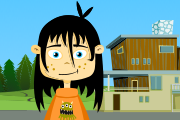### Salaby

Mathematics 1–2 Various exercises and games in geometry.### Teacher LED

Practise copying three-dimensional geometric shapes.### Matematikk 5-7

On Elevkanalen’s mathematics page, you can watch videos and learn about numbers, equations, geometry, measurements, statistics and probability.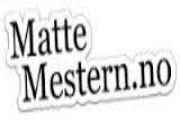### MatteMestern

Learn mathematics by watching, listening and doing exercises in Norwegian.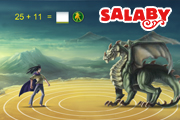### Salaby

Learn about geometry through exercises and games in Norwegian.### Teacher LED

Practise copying three-dimensional geometric shapes.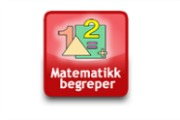### Tema morsmål

Illustrated list of mathematical terms in English and Norwegian (bokmål).### Tema morsmål

Illustrated list of mathematical terms in English and Norwegian (nynorsk).### FBU

Get an overview of words and concepts from maths in Norwegian and in your language, with illustrations.### Geometri

Watch videos about geometry. From the project Fleksibel opplæring.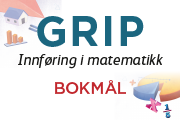### GRIP

Book with texts and exercises in counting, addition, subtraction, multiplication, division, positive and negative numbers, fractions, percentages, equations.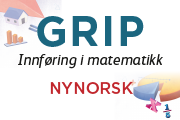### GRIP

Book with texts and exercises in counting, addition, subtraction, multiplication, division, positive and negative numbers, fractions, percentages, equations.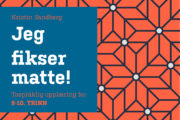### Jeg fikser matte

Mathematics for year 8-10. Overviews and explanations with mother-tongue support.### Kikora### MatteMestern

Learn mathematics by watching, listening and doing exercises in Norwegian.### Tema morsmål

Illustrated list of mathematical terms in English and Norwegian (bokmål).### Tema morsmål

Illustrated list of mathematical terms in English and Norwegian (nynorsk).### Tospråklig matematikk 8-10

On Elevkanalen’s bilingual mathematics page, you can watch lots of videos. You can learn about numbers, fractions and percentages, algebra, equations and formulas, measurements, geometry, graphs and functions, statistics, probability and economics. In the menu, you can choose between Norwegian (Bokmål), Arabic, Dari, Pashto, Kurmanji and Tigrinya.